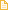## Posts tagged ‘local’

### Article: Triadic closure in two-mode networks: Redefining the global and local clustering coefficientsA paper called “Triadic closure in two-mode networks: Redefining the global and local clustering coefficients” that I have authored will be published in the special issue of Social Networks on two-mode networks (2012).

As the vast majority of network measures are defined for one-mode networks, two-mode networks often have to be projected onto one-mode networks to be analyzed. A number of issues arise in this transformation process, especially when analyzing ties among nodes’ contacts. For example, the values attained by the global and local clustering coefficients on projected random two-mode networks deviate from the expected values in corresponding classical one-mode networks. Moreover, both the local clustering coefficient and constraint (structural holes) are inversely associated to nodes’ two-mode degree. To overcome these issues, this paper proposes redefinitions of the clustering coefficients for two-mode networks.

### Degree Centrality and Variation in Tie WeightsA central metric in network research is the number of ties each node has, degree. Degree has been generalised to weighted networks as the sum of tie weights (Barrat et al., 2004), and as a function of the number of ties and the sum of their weights (Opsahl et al., 2010). However, all these measures are insensitive to variation in the tie weights. As such, the two nodes in this diagram would always have the same degree score. This post showcases a new measure that uses a tuning parameter to control whether variation should be taken favourable or discount the degree centrality score of a focal node.

### Article: Node centrality in weighted networks: Generalizing degree and shortest pathsA paper called “Node centrality in weighted networks: Generalizing degree and shortest paths” that I have co-authored will be published in Social Networks. Ties often have a strength naturally associated with them that differentiate them from each other. Tie strength has been operationalized as weights. A few network measures have been proposed for weighted networks, including three common measures of node centrality: degree, closeness, and betweenness. However, these generalizations have solely focused on tie weights, and not on the number of ties, which was the central component of the original measures. This paper proposes generalizations that combine both these aspects. We illustrate the benefits of this approach by applying one of them to Freeman’s EIES dataset.

### Closeness centrality in networks with disconnected componentsA key node centrality measure in networks is closeness centrality (Freeman, 1978; Wasserman and Faust, 1994). It is defined as the inverse of farness, which in turn, is the sum of distances to all other nodes. As the distance between nodes in disconnected components of a network is infinite, this measure cannot be applied to networks with disconnected components (Opsahl et al., 2010; Wasserman and Faust, 1994). This post highlights a possible work-around, which allows the measure to be applied to these networks and at the same time maintain the original idea behind the measure.

### Local clustering coefficient for two-mode networksSimilar to the motivation of the global clustering coefficient that I proposed in Clustering in two-mode networks, the local clustering coefficient is biased if applied to a projection of a two-mode network. It is biased in the sense that the randomly expected value is not obtained on the projection of a random two-mode network. To overcome this methodological bias, I redefine the local clustering coefficient for two-mode networks. The new coefficient is a mix between the global clustering coefficient for two-mode networks and Barrat’s (2004) local coefficient for a weighted one-mode network. The coefficient is tested on Davis’ (1940) Southern Women dataset.

The content of this post has been integrated in the tnet manual, see Clustering in Two-mode Networks.

### Similarity between node degree and node strengthThis post explores the relationship between node degree and node strength in an online social network. In the online social network, heterogeneity in nodes’ average tie weight across different levels of degree had been reported. Although degree and average tie weight are significantly correlated, this post argues for the similarity of degree and node strength. In particular, high pair-wise correlation between degree and strength is found. In addition, power-law exponents of degree distributions and strength distribution are reported. The exponents are strikingly similar, in fact, they are almost identical.

### Clustering in two-mode networksMany network dataset are by definition two-mode networks. Yet, few network measures can be directly applied to them. Therefore, two-mode networks are often projected onto one-mode networks by selecting a node set and linking two nodes if they were connected to common nodes in the two-mode network. This process has a major impact on the level of clustering in the network. If three or more nodes are connected to a common node in the two-mode network, the nodes form a fully-connected clique consisting of one or more triangles in the one-mode projection. Moreover, it produces a number of modeling issues. For example, even a one-mode projection of a random two-mode network with same number of nodes and ties will have a higher clustering coefficient than the randomly expected value. This post represents an attempt to overcome this issue by redefining the clustering coefficient so that it can be calculated directly on the two-mode structure. I illustrate the benefits of such an approach by applying it to two-mode networks from four different domains: event attendance, scientific collaboration, interlocking directorates, and online communication.

The content of this post has been integrated in the tnet manual, see Clustering in Two-mode Networks.